# TypeScript实现八大排序与搜索算法

## 排序算法

### 冒泡排序

#### 实现思路

• 声明一个函数其接受两个参数：待排序数组 & 比对函数
• 第一层循环i：从待排序数组的0号元素开始遍历数组，遍历到最后一位，用于控制数组需要经过多少轮排序
• 第二层循环j：从数组的0号元素开始遍历，遍历至数组的倒数第二位元素再减去第一层循环i。
• 比较大小，在第二层循环中，将当前遍历到的元素和其下一个元素比较大小，如果 j > j + 1就交换两个元素的位置。

#### 实现代码

• 为了函数的可扩展性，我们需要实现一个默认的比对函数，此比对函数作用域本文中的所有排序方法
``````export function defaultCompare<T>(a: T, b: T): number {
if (a === b) {
return Compare.EQUALS;
} else if (a > b) {
return Compare.BIGGER_THAN;
} else {
return Compare.LESS_THAN;
}
}

// 枚举类：定义比对返回值
export enum Compare {
LESS_THAN = -1,
BIGGER_THAN = 1,
EQUALS = 0
}

• 在类中声明需要的变量，并在构造器中声明需要传的参数类型，此处适用于本文实现的所有函数
``````    /**
* 排序算法
* @param array 需要进行排序的数组
* @param compareFn 比对函数
*/
constructor(private array: T[] = [], private compareFn: ICompareFunction<T> = defaultCompare) {}

• 实现冒泡排序
``````    // 冒泡排序
bubbleSort(): void {
// 获取数组长度
const { length } = this.array;
for (let i = 0; i < length; i++) {
// 从数组的0号元素遍历到数组的倒数第2号元素，然后减去外层已经遍历的轮数
for (let j = 0; j < length - 1 - i; j++) {
// 如果j > j + 1位置的元素就交换他们两个元素的位置
if (this.compareFn(this.array[j], this.array[j + 1]) === Compare.BIGGER_THAN) {
this.swap(this.array, j, j + 1);
}
}
}
}

#### 编写测试代码

``````const array = [12, 6, 3, 4, 1, 7];
const sort = new Sort(array);
sort.bubbleSort();
console.log(array.join());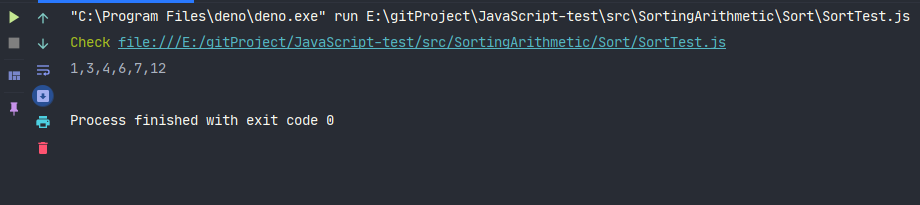### 选择排序

#### 实现思路

• 声明一个辅助变量:indexMin用于存储数组中的最小值
• 第一层循环i，从数组的0号元素遍历到数组的倒数第二位。indexMin默认赋值为i，用于控制轮数
• 第二层循环j，从数组的i号元素遍历到数组的末尾，用于寻找数组的最小值，如果indexMin位置的元素大于j号位置的元素就覆盖indxMin的值为j
• 在第二层循环结束后，比较i与indexMin是否相等，如果不相等就交换两个元素的位置。

#### 实现代码

``````    // 选择排序
selectionSort(): void {
const { length } = this.array;
// 声明一个变量用于存储最小元素的位置
let indexMin = 0;
for (let i = 0; i < length; i++) {
// 初始值为外层循环当前遍历到的位置i
indexMin = i;
for (let j = i; j < length; j++) {
// 如果当前遍历到元素小于indexMin位置的元素，就将当前遍历到的位置j赋值给indexMin
if (this.compareFn(this.array[indexMin], this.array[j]) === Compare.BIGGER_THAN) {
indexMin = j;
}
}
if (i !== indexMin) {
this.swap(this.array, i, indexMin);
}
}
}

#### 编写测试代码

``````const array = [12, 6, 3, 4, 1, 7];
const sort = new Sort(array);
// console.log(result.join());

sort.selectionSort();
console.log(array.join());### 插入排序

#### 实现思路

• 第一层循环i：从数组的1号元素开始，遍历到数组末尾，因为我们会先假设0号元素已经排好序，所以从1号元素开始。
• 用一个临时变量`temp`存储当前i号位置的元素，用一个变量`j`存储i
• while循环: `j > 0``j - 1`位置的元素大于`temp`，就把`j `位置的值设置为`j - 1` 位置的值，最后j--，继续下一轮遍历。
• while循环结束后，将`temp`放到正确的位置`array[ j ]`

#### 实现代码

``````    // 插入排序
insertionSort(array: T[] = this.array): void {
const { length } = array;
let temp;
// 假设0号元素已经排好序，从1号元素开始遍历数组
for (let i = 1; i < length; i++) {
// 声明辅助变量存储当前i的位置以及其对应的值
let j = i;
temp = array[i];
// j大于0且j-1位置的元素大于i号位置的元素就把j-1处的值移动到j处，最后j--
while (j > 0 && this.compareFn(array[j - 1], temp) === Compare.BIGGER_THAN) {
array[j] = array[j - 1];
j--;
}
// 将temp放到正确的位置
array[j] = temp;
}
}

#### 编写测试代码

``````const array = [12, 6, 3, 4, 1, 7];
const sort = new Sort(array);
sort.insertionSort(array);
console.log(array.join());### 归并排序

#### 实现思路

• 递归终止条件：由于是递归，所以我们需要先设立递归终止条件，当数组的长度大于1时就进行归并排序。
• 获取数组的中间值: 我们通过数组的中间值来切割数组，将其分成左、右两部分。对左右两部分继续执行分割
• 合并数组: 我们将数组分割完后，对小数组进行排序，然后将其合并成大数组并返回。

• 合并函数接收两个参数：左、右数组
• 声明三个辅助变量: `i, j, result`，分别表示左、右数组的指针以及存储合并后的数组
• 如果`i < left.length && j < right.length`，代表指针数组尚未排序完，因此执行下述逻辑
• 如果`lef[i] < right[j]`，就往`result`中放`left[i]`的值随后i++，否则就放right[j]的值随后j++
• 最后，将`left``right`数组的剩余项添加进`result`

#### 实现代码

``````    // 归并排序
mergeSort(array: T[] = this.array): T[] {
if (array.length > 1) {
const { length } = array;
// 获取中间值
const middle = Math.floor(length / 2);
// 递归填充左右数组
const left = this.mergeSort(array.slice(0, middle));
const right = this.mergeSort(array.slice(middle, length));
// 合并左右数组
array = this.merge(left, right);
}
return array;
}

private merge(left: T[], right: T[]) {
let i = 0;
let j = 0;
const result: T[] = [];
while (i < left.length && j < right.length) {
// 如果left[i] < right[j]将left[i]加进result中，随后i自增，否则把right[j]加进result中，随后j自增
result.push(this.compareFn(left[i], right[j]) === Compare.LESS_THAN ? left[i++] : right[j++]);
}
// 将left或right数组的剩余项添加进result中
return result.concat(i < left.length ? left.slice(i) : right.slice(j));
}

#### 编写测试代码

``````const array = [12, 6, 3, 4, 1, 7];
const sort = new Sort(array);
const result = sort.mergeSort();
console.log(result.join());### 快速排序

#### 实现思路

• 主函数（quickSort），调用排序函数，返回排序函数最终排好的数组。

• 排序函数（quick），接收3个参数: 待排序数组（array）、数组的左边界（left）、数组的右边界（right）

• 声明一个辅助变量`index`，用于存储分割子数组的的位置。
• 确立递归终止条件：`array < 1` ，如果`array.length > 1`，就执行下述操作：

执行划分函数，计算划分点，将得到的值赋值给`index`； 如果`left > index - 1`，代表子数组中存在较小值的元素，从数组的`left边界``index-1`边界递归执行排序函数； 如果`index < right`，代表子数组存在较大值的元素，从数组的`index``right`边界递归执行排序函数；

• 划分函数（partition），与排序函数一样，它也接收3个参数。

• 从数组中选择一个主元`pivot`，选择数组的中间值
• 声明两个指针：`i, j`，分别指向走遍数组的第一个值和右边数组的第一个值。
• 如果`i <= j`，则代表left指针和right指针没有相互交错，则执行下述划分操作：

移动left指针直至找到一个比主元大的元素，即`array[i] > pivot`； 移动right指针直至找到一个比主元小的元素，即`array[j] < pivot`； 当左指针指向的元素比主元大且右指针指向的元素比主元小，并且左指针索引没有右指针索引大时就交换i号和j号元素的位置，随后移动两个指针； 最后，划分结束，返回i的值；

#### 实现代码

``````    /**
*
* @param array 待排序数组
* @param left 左边界
* @param right 右边界
* @private
*/
private quick(array: T[], left: number, right: number) {
// 该变量用于将子数组分离为较小值数组和较大值数组
let index;
if (array.length > 1) {
// 对给定子数组执行划分操作，得到正确的index
index = this.partition(array, left, right);
// 如果子数组存在较小值的元素，则对该数组重复这个过程
if (left < index - 1) {
this.quick(array, left, index - 1);
}
// 如果子数组存在较大值的元素，也对该数组重复这个过程
if (index < right) {
this.quick(array, index, right);
}
}
return array;
}

// 划分函数
private partition(array: T[], left: number, right: number): number {
// 从数组中选择一个值做主元，此处选择数组的中间值
const pivot = array[Math.floor((right + left) / 2)];
// 创建数组引用，分别指向左边数组的第一个值和右边数组的第一个值
let i = left;
let j = right;

// left指针和right指针没有相互交错，就执行划分操作
while (i <= j) {
// 移动left指针直至找到一个比主元大的元素
while (this.compareFn(array[i], pivot) === Compare.LESS_THAN) {
i++;
}

// 移动right指针直至找到一个比主元小的元素
while (this.compareFn(array[j], pivot) === Compare.BIGGER_THAN) {
j--;
}

// 当左指针指向的元素比主元大且右指针指向的元素比主元小，并且左指针索引没有右指针索引大时就交换i和j号元素的位置，随后移动两个指针
if (i <= j) {
this.swap(array, i, j);
i++;
j--;
}
}
// 划分结束，返回左指针索引
return i;
}

#### 编写测试代码

``````const array = [12, 6, 3, 4, 1, 7];
const sort = new Sort(array);
const result = sort.quickSort();
console.log(result.join());### 计数排序

#### 实现思路

• 找到要排序数组的最大值
• 以上一步找到的最大值+1为长度创建一个计数数组
• 遍历要排序的数组，以当前遍历的元素为索引，寻找计数数组中对应的位置将其初始化为0，如果此处位置有相同元素的话就自增
• 遍历计数数组，判断当前遍历到的元素是否大于0，如果大于0就取当前遍历到的索引，替换待排序数组中的元素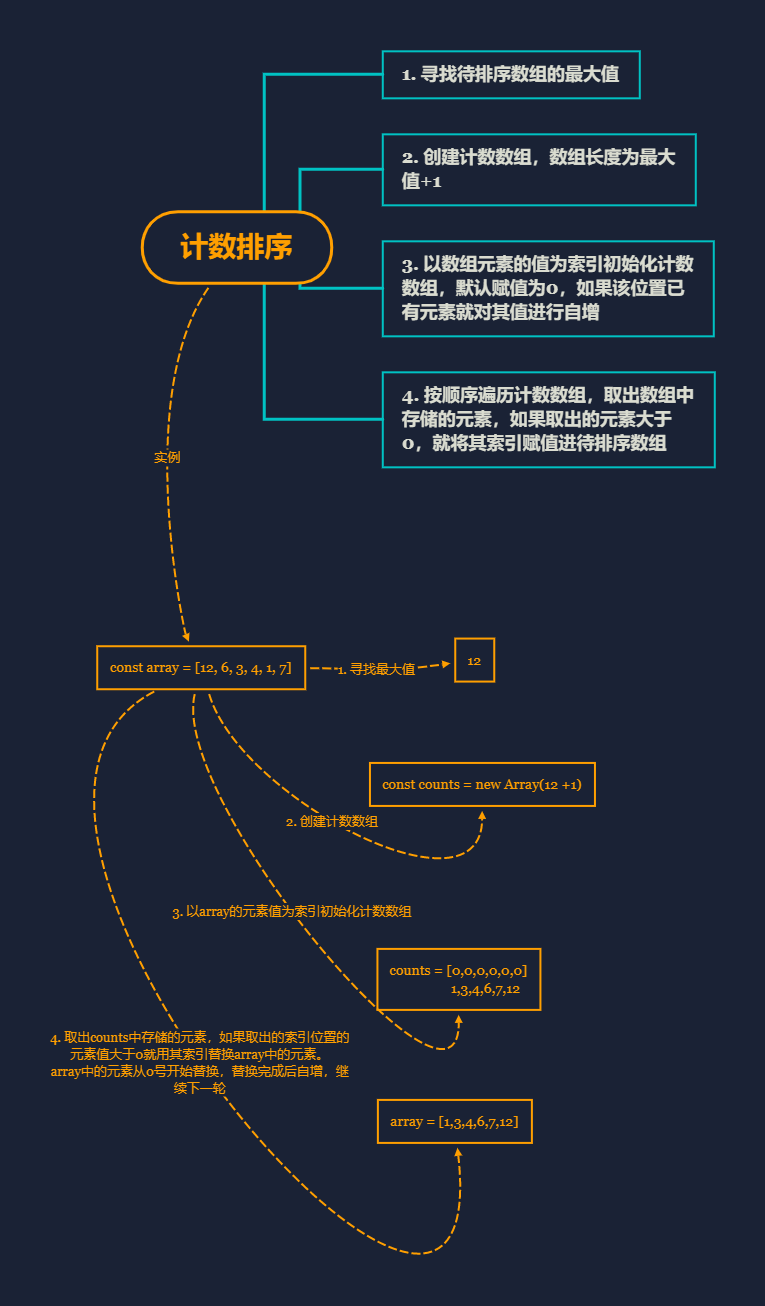#### 实现代码

``````    countingSort(array: number[]): number[] {
// 待排序数组为空或只有一个元素则不用排序
if (array.length < 2) {
return array;
}
// 找到待排序数组中的最大值
const maxValue = this.findMaxValue(array);

// 创建计数数组，数组长度为待排序数组的最大值+1
const counts = new Array(maxValue + 1);
// 遍历待排序数组，为计数数组赋值
array.forEach((element) => {
// 以当前遍历到的元素值为索引将对应位置元素值初始化为0
if (!counts[element]) {
counts[element] = 0;
}
// 当前位置的值进行自增，顺便应对数组中有重复值的情况，有重复值时当前位置的值必定大于1
counts[element]++;
});

// 声明一个变量用于数组最终排序
let sortedIndex = 0;
// 遍历计数数组，根据计数数组的元素位置对待排序数组进行排序
counts.forEach((count, i) => {
// 如果当前遍历到的元素值大于0，则执行替换操作进行排序
while (count > 0) {
// 将当前元素索引赋值给array的sortedIndex号元素，随后sortedIndex自增
array[sortedIndex++] = i;
// 当前元素值自减，如果其值大于1，证明此处有重复元素，那么我们就继续执行while循环
count--;
}
});
// 最后，排序完成，返回排序好的数组
return array;
}

#### 编写测试代码

``````const array = [12, 6, 3, 4, 1, 7];
const sort = new Sort(array);
const result = sort.countingSort(array);
console.log(result.join());### 桶排序

#### 实现思路

• 创建桶，并将桶分布到不同的桶中
• 对每个桶中的元素执行排序算法并将所有桶合并成排序好后的结果数组

• 声明创建桶函数（createBuckets），接收两个参数：待排序数组（`array`），桶大小（`bucketSize`
• 计算`array`中的最大值（`maxValue`）和最小值（`minValue`
• 计算每个需要的桶数量，公式为：`(maxValue - minValue) / bucketSize)+1`
• 声明一个二维数组`buckets`，用于存放所有桶
• 根据桶数量，初始化每个桶
• 遍历`array`，将每个元素分布到桶中
• 计算需要将元素放到哪个桶中(bucketIndex)，公式为：`(array[i] - minValue) / bucketSize`
• 将元素与放进合适的桶中，即`buckets[bucketIndex].push(array[i])`
• 将桶返回

• 创建排序桶函数（sortBuckets），接收一个参数: 桶`buckets`
• 需要一个辅助数组`sortedArray`，用于存放排序好的结果数组
• 遍历`sortedArray`
• 如果当前桶内存储的桶`buckets[i]`不为null，就对其执行插入排序
• 将排序好的桶`buckets[i]`解构后放进`sortedArray`
• 最后，返回`sortedArray`

#### 实现代码

``````    // 桶排序
bucketSort(array: number[], bucketSize = 5): T[] | number[] {
if (array.length < 2) {
return array;
}
// 创建桶，对桶进行排序
return this.sortBuckets(<[][]>this.createBuckets(array, bucketSize));
}

/**
* 创建桶
* @param array 待排序数组
* @param bucketSize 桶大小，即每个桶里的元素数量
*
* @return 二维数组类型的桶
*/
private createBuckets = (array: number[], bucketSize: number): number[][] => {
// 计算数组最大值与最小值
let minValue = array;
let maxValue = array;
for (let i = 1; i < array.length; i++) {
if (array[i] < minValue) {
minValue = array[i];
} else if (array[i] > maxValue) {
maxValue = array[i];
}
}

// 计算需要的桶数量，公式为: 数组最大值与最小值的差值与桶大小进行除法运算
const bucketCount = Math.floor((maxValue - minValue) / bucketSize) + 1;
// 用于存放桶的二维数组
const buckets: number[][] = [];

// 计算出桶数量后，初始化每个桶
for (let i = 0; i < bucketCount; i++) {
buckets[i] = [];
}

// 遍历数组，将每个元素分布到桶中
for (let i = 0; i < array.length; i++) {
// 计算需要将元素放到哪个桶中，公式为: 当前遍历到的元素值与数组的最小值的差值与桶大小进行除法运算
const bucketIndex: number = Math.floor((array[i] - minValue) / bucketSize);
// 将元素放进合适的桶中
buckets[bucketIndex].push(array[i]);
}
// 将桶返回
return buckets;
};

// 对每个桶进行排序
private sortBuckets(buckets: T[][]): T[] {
const sortedArray: T[] = [];
for (let i = 0; i < buckets.length; i++) {
if (buckets[i] != null) {
// 调用插入排序
this.insertionSort(buckets[i]);
// 将排序好的桶取出来，放进sortedArray中
sortedArray.push(...buckets[i]);
}
}
return sortedArray;
}

#### 编写测试代码

``````const array = [12, 5, 6, 7, 8, 9, 11, 3, 4, 19];
const sort = new Sort(array);
const result = sort.bucketSort(array);
console.log(result.join());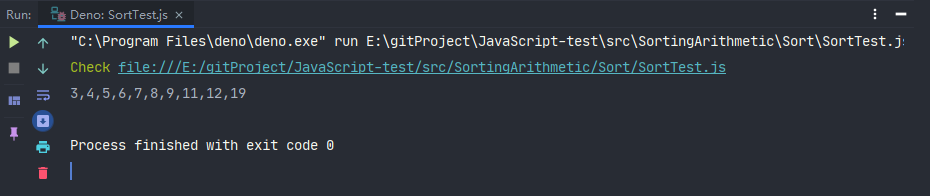### 基数排序

#### 实现思路

• 创建基数排序函数（radixSort），接受2个参数：待排序数组`array`，基数`radixBash`

• 首先，获取`array`的最大值`maxValue`和最小值`minValue`

• 声明一个辅助变量`significantDigit`，用于存储当前有效数字，默认从最后一位有效数字，即1

• 计算有效位，公式为：`(maxValue - minValue) / significantDigit`，计算出的值如果大于等于`1`执行下述逻辑：

• 以当前有效位作为参数调用`singnificantDigit`函数对数组进行排序
• 当前有效数字*基数，继续执行上述过程
• 最后，执行完成返回排序好多的数组

• 创建`countingSortForRadix`函数，接受4个参数：待排序数组`array`，基数`radixBase`、有效位`significantDigit`、数组的最小值`minValue`

• 声明桶索引`bucketsIndex`以及桶`buckets`以及辅助数组`aux`

• 通过`radixBase`来初始化桶，默认初始化为0

• 遍历`array`，基于有效位计算桶索引执行计数排序

• 计算桶索引，公式为：`((array[i] - minValue) / significantDigt) % radixBase`
• 根据桶索引，执行计数操作，即`buckets[bucketsIndex++]`

• `buckets[i]`等于`buckets[i]` 加上`buckrts[i - 1]`的值
• 计数完成，遍历`array`将值移回原始数组中，用aux辅助数组来存储

• 计算当前元素的桶索引`bucketsIndex`，公式为：`((array[i] - minValue) / significantDigit) % radixBase`
• 对当前桶索引内的元素执行自减操作，得到其在数组中的正确位置`index`
• 计算出索引后，在aux中的对应位置存储当前遍历到的元素
• 最后排序完成，返回axu。

#### 实现代码

``````    /**
* 基数排序
* @param array 待排序数组
*/
if (array.length < 2) {
return array;
}
// 获取数组的最小值和最大值
const minValue = this.findMinValue(array);
const maxValue = this.findMaxValue(array);
// 当前有效数字,默认会从最后一位有效数字开始排序
let significantDigit = 1;

/**
* 计算有效位
* 最大值和最小值的差与有效数字进行除法运算，其值大于等于1就代表还有待排序的有效位
*/
while ((maxValue - minValue) / significantDigit >= 1) {
// 以当前有效位为参数对数组进行排序
// 当前有效数字乘以基数，继续执行while循环进行基数排序
}
return array;
}

/**
* 基于有效位进行排序
* @param array 待排序数组
* @param significantDigit 有效位
* @param minValue 待排序数组的最小值
*/
private countingSortForRadix = (array: number[], radixBase: number, significantDigit: number, minValue: number) => {
// 声明桶索引以及桶
let bucketsIndex;
const buckets = [];
// 辅助数组，用于计数完成的值移动会原数组
const aux = [];
// 通过基数来初始化桶
for (let i = 0; i < radixBase; i++) {
buckets[i] = 0;
}

// 遍历待排序数组，基于有效位计算桶索引执行计数排序
for (let i = 0; i < array.length; i++) {
// 计算桶索引
bucketsIndex = Math.floor(((array[i] - minValue) / significantDigit) % radixBase);
// 执行计数操作
buckets[bucketsIndex]++;
}

// 计算累积结果得到正确的计数值
for (let i = 1; i < radixBase; i++) {
buckets[i] = buckets[i] + buckets[i - 1];
}

// 计数完成，将值移回原始数组中,用aux辅助数组来存储
for (let i = array.length - 1; i >= 0; i--) {
// 计算当前元素的桶索引
bucketsIndex = Math.floor(((array[i] - minValue) / significantDigit) % radixBase);
// 对当前桶索引内的元素执行自减操作,得到其在数组中的正确的位置
const index = --buckets[bucketsIndex];
// 计算出索引后，在aux中的对应位置存储当前遍历到的元素
aux[index] = array[i];
}
console.log(aux);
return aux;
};

#### 编写测试代码

``````const array = [12, 5, 6, 7, 8, 9, 11, 3, 4, 19];
const sort = new Sort(array);
console.log(result.join());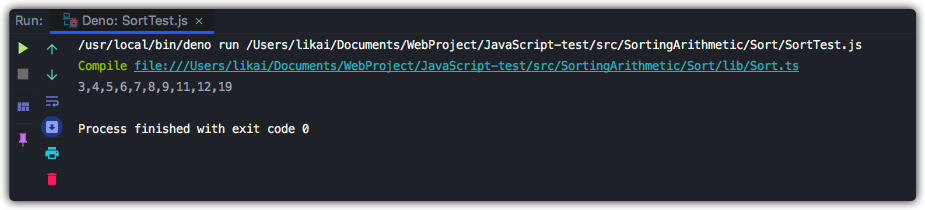## 搜索算法

### 顺序搜索

#### 实现代码

``````    linearSearch(): number | null {
for (let i = 0; i < this.array.length; i++) {
if (this.array[i] === this.target) {
return i;
}
}
return null;
}

#### 编写测试代码

``````const array = [7, 8, 1, 2, 3, 5, 12, 13, 16, 19];
const searchArithmetic = new SearchArithmetic(array, 7);
const mid = searchArithmetic.linearSearch();
console.log(mid);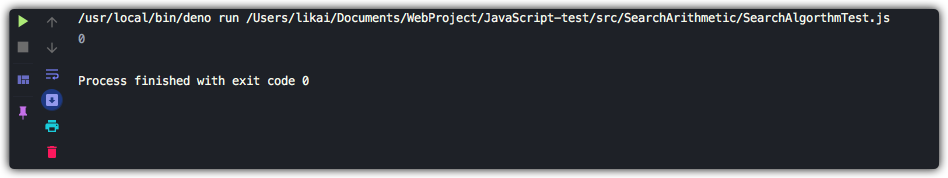### 二分搜索

#### 实现思路

• 选择数组的中间值
• 如果选中值是待搜索值，那么算法执行完毕
• 如果待搜索值比选中值要小，则返回步骤1并在选中值左边的子数组中寻找（较小）
• 如果待搜索值比选中值要大，则返回步骤1并在选中值右边的子数组中寻找（较大）

#### 实现代码

``````    binarySearch(): number | null {
// 对数组进行排序
this.sort.quickSort();
// 设置指针作为数组边界
let low = 0;
let high = this.array.length - 1;
while (low <= high) {
// 获取数组中间值
const mid = Math.floor((low + high) / 2);
// 获取数组的中间值
const element = this.array[mid];
// 如果数组中间值小于目标值，low+1,向其右边继续找
if (this.compareFn(element, this.target) === Compare.LESS_THAN) {
low = mid + 1;
} else if (this.compareFn(element, this.target) === Compare.BIGGER_THAN) {
// 如果中间值大于目标值,向其左边继续找
high = mid - 1;
} else {
// 中间值等于目标值，元素找到，返回mid即当前元素在数组的位置
return mid;
}
}
// 未找到，返回null
return null;
}

#### 编写测试代码

``````const array = [7, 8, 1, 2, 3, 5, 12, 13, 16, 19];
const searchArithmetic = new SearchArithmetic(array, 7);
const mid = searchArithmetic.binarySearch();
console.log(mid);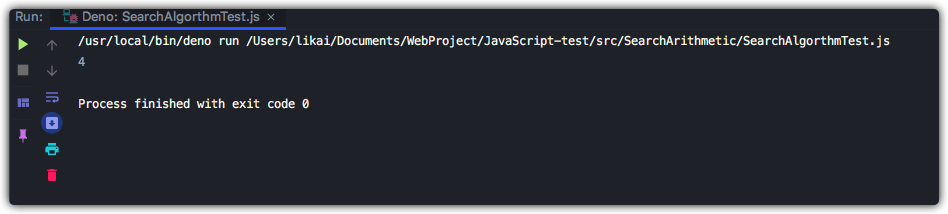### 内插搜索

#### 实现思路

• 使用`position`公式选中一个值
• 如果待搜索值比选中值要小，则返回步骤1并在选中值左边的子数组中寻找（较小）
• 如果待搜索值比选中值要大，则返回步骤1并在选中值右边的子数组中寻找（较大）

#### 实现代码

``````    /**
* 内插搜索
*  二分查找的优化，通过特定算法计算delta和position的值优化掉二分查找的寻找中间值做法
* @param equalsFn 校验两个值是否相等函数
* @param diffFn 计算两个数的差值函数
*/
interpolationSearch(equalsFn = defaultEquals, diffFn: IDiffFunction<T> = defaultDiff): number | null {
// 排序
this.sort.quickSort();
// 获取数组程度
const { length } = this.array;
// 定义指针，确定数组边界
let low = 0;
let high = length - 1;
// 声明position，用于公式
let position = -1;
let delta = -1;
// 目标值大于等于数组的low边界值且目标值小于等于high边界值就执行循环里的内容
while (low <= high && biggerEquals(this.target, this.array[low], this.compareFn) && lesserEquals(this.target, this.array[high], this.compareFn)) {
// 目标值与array的low边界的值做差
// 与array的high边界的值和low边界的值做差
// 最后将二者得出的值做除法运算，计算出delta值
delta = diffFn(this.target, this.array[low]) / diffFn(this.array[high], this.array[low]);
// 计算比较值的位置
position = low + Math.floor((high - low) * delta);
// 如果比较值位置的元素等于目标值，则返回当前索引
if (equalsFn(this.array[position], this.target)) {
return position;
}
// 如果比较值位置的元素小于目标值，则向其右边继续找
if (this.compareFn(this.array[position], this.target) === Compare.LESS_THAN) {
low = position + 1;
} else {
// 如果比较值位置的元素大于目标值，则向其左边继续查找
high = position - 1;
}
}
// 未找到
return null;
}

#### 编写测试代码

``````const array = [7, 8, 1, 2, 3, 5, 12, 13, 16, 19];
const searchArithmetic = new SearchArithmetic(array, 7);
const mid = searchArithmetic.interpolationSearch();
console.log(mid);## 随机算法

### Fisher-Yates算法

#### 实现代码

``````export class ShuffleArithmetic<T> {
constructor(private array: T[]) {}
// Fisher-Yates随机算法
fisherYates(): T[] {
// 从数组的最后一位向前遍历数组
for (let i = this.array.length - 1; i > 0; i--) {
// 计算随机位置,用一个随机数与i+1相加,得出的随机位置一定比当前位置小
const randomIndex = Math.floor(Math.random() * (i + 1));
// 交换当前位置的元素和随机位置的元素
this.swap(i, randomIndex);
}

return this.array;
}

/**
* 交换数组的元素
* @param a
* @param b
* @private
*/
private swap(a: number, b: number) {
const temp: T = this.array[a];
this.array[a] = this.array[b];
this.array[b] = temp;
}
}

#### 编写测试代码

``````import { ShuffleArithmetic } from "./lib/ShuffleArithmetic.ts";

const array = [4, 5, 6, 1, 2, 3, 4, 5, 8, 9, 0, 11, 22, 41];
const shuffleArithmetic = new ShuffleArithmetic(array);
shuffleArithmetic.fisherYates();
console.log("随机排序后的数组元素", array.join());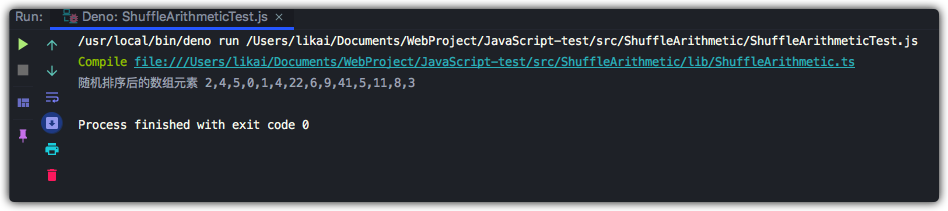## 写在最后

• 文中如有错误，欢迎在评论区指正，如果这篇文章帮到了你，欢迎点赞和关注😊
• 本文首发于掘金，未经许可禁止转载💌